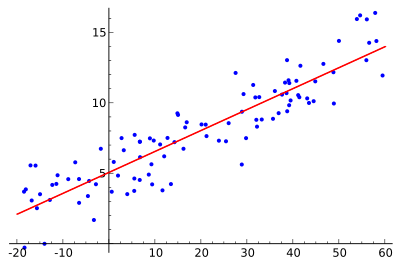# Linear regression facts for kids

Kids Encyclopedia FactsThe idea is to find the red curve, the blue points are actual samples. With linear regression all points can be connected using a single, straight line. This example uses simple linear regression, where the square of the distance between the red line and each sample point is minimized.

Linear regression is a way to explain the relationship between a dependent variable and one or more explanatory variables using a straight line. It is a special case of regression analysis.

Linear regression was the first type of regression analysis to be studied rigorously. This is because models which depend linearly on their unknown parameters are easier to fit than models which are non-linearly related to their parameters. What is more, the statistical properties of the resulting estimators are easier to determine.

Linear regression has many practical uses. Most applications fall into one of the following two broad categories:

• Linear regression can be used to fit a predictive model to a set of observed values (data). This is useful, if the goal is prediction, or forecasting, or reduction. After developing such a model, if an additional value of X is then given without its accompanying value of y, the fitted model can be used to make a prediction of the value of y.
• Given a variable y and a number of variables X1, ..., Xp that may be related to y, linear regression analysis can be applied to quantify the strength of the relationship between y and the Xj, to assess which Xj has no relationship with y at all, and to identify which subsets of the Xj contain redundant information about y.

Linear regression models try to make the vertical distance between the line and the data points (e.g the residuals) as small as possible. This is called "fitting the line to the data." Often, linear regression models try to minimize the sum of the squares of the residuals (least squares), but other ways of fitting exist. They include minimizing the "lack of fit" in some other norm (as with least absolute deviations regression), or minimizing a penalized version of the least squares loss function as in ridge regression. The least squares approach can also be used to fit models that are not linear. As outlined above, the terms "least squares" and "linear model" are closely linked, but they are not synonyms.

## Usage

### Economics

Linear regression is the main analytical tool in economics. For example, it is used to guess consumption spending, fixed investment spending, inventory investment, purchases of a country's exports, spending on imports, the demand to hold liquid assets, labor demand, and labor supply.

## Images for kidsLinear regression Facts for Kids. Kiddle Encyclopedia.